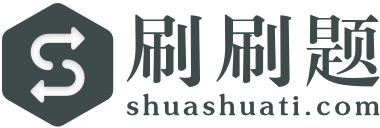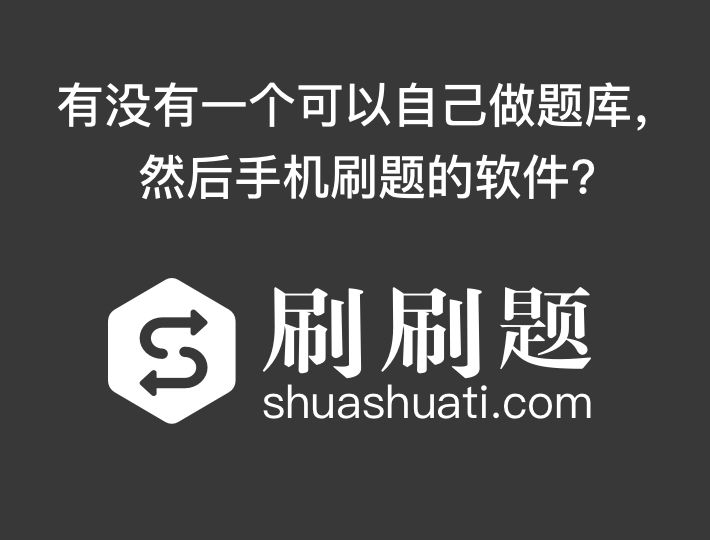【简答题】请参照实验报告模版撰写实验报告,并按时提交。 实验报告模版.docA.
0101
B.
1000
C.
0111
D.
1101

A.

B.

C.

D.

E.

A.

B.

C.

D.

E.

A.

B.

C.

D.

A.

B.

C.

D.

a- b题共用题干)患者,女性,27岁,4小时前突然出现腹泻、伴呕吐排便10余次,呈水样便,量多,呕叶3次,初为胃内容物,后为水样。a.该患者最可能病因是:
A.

B.

C.

D.

E.

A.
10.24 修约为10.2
B.
1.25 修约为1.3
C.
7.051 修约为7.1
D.
0.31 修约为0.3

A.

B.

C.

D.

A.

B.

C.

D.

A.

B.

C.
10名以上的该县人大代表联名
D.
1/10以上的该县人大代表联名

A.

B.

C.

D.

E.

A.

B.

C.

D.

A.

B.

A.

B.

C.

A.

B.

C.

D.

E.

A.

B.

A.

B.

C.

D.

E.

A.

B.

C.

D.

A.

B.

C.

D.

A.

B.

C.

D.

E.

A.

B.

C.

D.

A.

B.

C.

D.

A.
2个输入/1个输出
B.
1个输入/2个输出
C.
2个输入/2个输出
D.
2个输入/3个输出
（49）的8421BCD是（ ）。（100087.0111.01010011）8421BCD对应的十进制数是（ ）
8421BCD码是恒权码
A.

B.

A.

B.

C.

D.

A.

B.

ABC 也叫主次因素分析法。
A.

B.

A.

B.

C.

D.

E.

3天前食后受凉,腹泻不止,日行6~7 次,水样便,腹微痛,纳呆恶心,小便短少,舌苔白厚腻,脉缓。辨证为
A.

B.

C.

D.

E.

A.

B.

C.

D.

A.

B.

A.
1 888.89
B.
66495.58
C.
85 000
D.
87 210
( )重奶油蛋糕的比重较海绵蛋糕面糊轻。
A.

B.

A.
B超
B.

C.

D.

E.
CT

A.

B.

C.

D.

A.

B.

C.

D.
１人で発表の内容を決めました。

A.

B.

C.

D.

A.

B.

C.

D.

A.

B.

A.

B.

A.

B.

C.

D.

A.
ほど
B.
まで
C.
さえ
D.
より

A.

B.

C.

D.

A.

B.

C.

D.

( )是人生观的核心。 A 人生态度 B 人生目的 C 人生价值 D 人生道路
A.

B.

C.

D.

A.
B.

A.

B.

C.

D.

A.

B.

A.

B.

C.

D.

A.

B.

A.

B.

C.
“两弹一星”研制成功有利于我国外交的发展
D.

A.

B.

C.

D.

A.

B.

A.

B.

A.

B.

C.

D.

A.

B.

A.
Intel
B.
IBM
C.
AMD
D.

（ ▲ ）T12中“”表示质量等级为级，在
A.

B.

“GB/T 31848—2015"中的“/T"表示的是（）。
A.

B.

C.

D.

A.

B.

C.

D.

A.

B.

C.

D.

A.

B.

C.

D.

1批计算机芯片从美国运到韩国，最佳的运输方式是（）
A.

B.

C.

D.

1批计算机芯片从美国运到韩国,最佳的运输方式是()
A.

B.

C.

D.

A.

B.

C.

D.

A.

B.

C.

D.

E.

A.

B.

C.

D.

E.

A.

B.

A.
\$
B.
\
C.
&
D.
#

A.

B.

C.

D.

A.

B.

C.

D.

E.

【例题·】A公司于2010年1月1日用货币资金从证券市场上购入B公司发行在外股份的25%，作为长期股权投资核算，并对B公司具有重大影响，实际支付价款569万元，另支付相关税费1万元，同日，B公司可辨认净资产的公允价值为2200万元，所有者权益总额为2100万元。A公司2010年1月1日应确认的损益为（　）万元。
A.
20
B.
0
C.
18
D.
－20

A.

B.

A.

B.

C.

D.

A.

B.

C.

D.

A.

B.

A.

B.

A.
22
B.
20
C.
18
D.
16
“日行一善，集腋成裘”，“见善则迁，有过则改”，讲的都是（ ）。
A.

B.

C.

D.

A.

B.

C.

D.

A.
150总吨及以上
B.
400总吨及以上
C.
800总吨及以上
D.
1000总吨及以上

A.

B.

A.

B.

2019年7月企业用银行存款替职工垫付水电费15000元，，则下列会计分录正确的是（）
A.

B.

C.

D.

A.

B.

《MARPOL73/78》附则I规定,凡400吨以上的( )( ),应装设经主管机关认可的滤油设备,以保证派入海中的含油混合物的含油量不超过15ppm。
A.

B.

C.

D.

A.

B.

C.

D.

E.

A.

B.

2016年,( )作为G20峰会的举办地,设计师以“西湖元素、浙江特色、江南韵味、大国风范”的理念,进行设计创作。
A.

B.

C.

D.

“集腋成裘”比喻积少成多，用在时间管理方面，是启发我们：生活中有很多零碎的时间很不为人注意，这些时间虽短，但却可以充分利用起来做一些事情
A.

B.